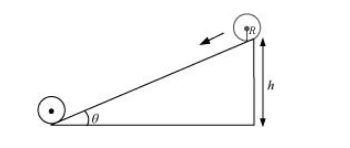# Prove the result that the velocity v of translation of a rolling body`
Question:

Prove the result that the velocity $v$ of translation of a rolling body (like a ring, disc, cylinder or sphere) at the bottom of an inclined plane of a height $h$ is given by $v^{2}=\frac{2 g h}{\left(1+k^{2} / R^{2}\right)}$.

Using dynamical consideration (i.e. by consideration of forces and torques). Note is the radius of gyration of the body about its symmetry axis, and R is the radius of the body. The body starts from rest at the top of the plane.

Solution:

A body rolling on an inclined plane of height h,is shown in the following figure:m = Mass of the body

K = Radius of gyration of the body

= Translational velocity of the body

=Height of the inclined plane

g = Acceleration due to gravity

Total energy at the top of the plane, $E_{1}=m g h$

Total energy at the bottom of the plane, $E_{\mathrm{b}}=\mathrm{KE}_{\mathrm{rot}}+\mathrm{KE}_{\mathrm{trans}}$

$=\frac{1}{2} I \omega^{2}+\frac{1}{2} m v^{2}$

But $I=m k^{2}$ and $\omega=\frac{v}{R}$

$\therefore E_{\mathrm{b}}=\frac{1}{2}\left(m k^{2}\right)\left(\frac{v^{2}}{R^{2}}\right)+\frac{1}{2} m v^{2}$

$=\frac{1}{2} m v^{2} \frac{k^{2}}{R^{2}}+\frac{1}{2} m v^{2}$

$=\frac{1}{2} m v^{2}\left(1+\frac{k^{2}}{R^{2}}\right)$

From the law of conservation of energy, we have

$E_{\mathrm{T}}=E_{\mathrm{b}}$

$m g h=\frac{1}{2} m v^{2}\left(1+\frac{k^{2}}{R^{2}}\right)$

$\therefore v=\frac{2 \mathrm{~g} h}{\left(1+k^{2} / R^{2}\right)}$

Hence, the given result is proved.